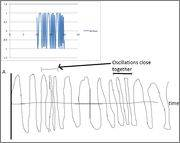# A formula for sinusoidal graphs of this form?

Hey all

I am trying to generate a graph of a sinusoidally oscillation against time. However, the time itself is passing sinusodally, i.e time flows faster at some points and slower at others. I'm doing this as I need test data for a program I've written to decode real world data I'm generating which comes in this format.

Here is an excel graph of the closest I've come (amplitude = sin(time*sin(time))), however this is not quite correct, as it goes off as time increases (as the time between oscillations get closer and closer. but between t=10 and t =20 you can get a good idea of what I'm after.)

I have also stuck a very crude sketch of the type of output I desire. What's the mathematical form of this?

C xBTW, in the excel version, the only reason my graph doesnt reach an amplitude of 1 consistently is due to a low sampling rate.

HallsofIvy
Homework Helper
Something like $x(t)= A sin(\omega (sin(kt))$ should work.

jbriggs444
Homework Helper
Something like $x(t)= A sin(\omega (sin(kt))$ should work.

I think I first encountered this problem in 1975 of so at the University of Illinois. An older student, Rob Kolstad, was trying to do a graph of a frequency modulation wave form for use in a course he was running on a CAI system named PLATO. He was trying to do much the same thing, modulating a sine function into the frequency of a sine wave carrier.

He was smart enough to realize that you have to add a constant offset to the inner sin function so that it's always positive. But, as I recall, that still gives an answer that's wrong.

You want to use the _integral_ of the function that you are trying to modulate onto the carrier. That way the frequency of the function that you are graphing varies as the derivitive of that integral -- i.e. as the signal that you're trying to carry.

I like Serena
Homework Helper
Welcome to PF, Carla17!Your inner time function should increase monotonously.
##\sin(time)## does not do that.

Try for instance ##x + \sin(x)## instead.
That one will just be increasing monotonously.

The complete function would be something like:
$$amplitude = \sin(\omega (t + \frac 1 k \sin(kt)))$$
where
##\omega## is the average angular frequency of the high frequency wave,
##k## is the angular frequency of the compression/decompression cycle,
##\frac 1 k## is the compensating factor to make the time just increasing, you may want to dampen it with another factor.​

Here's an example: http://m.wolframalpha.com/input/?i=sin(11*(t+++(1/3)*sin(2*t))&x=0&y=0
It graphs ##\sin(11 (t + \frac 1 3 \sin(2t)))##.

Last edited:
Why don't you guys use the obvious form

$$f(t)= A\sin\left( \left( \frac{\omega_\text{max}-\omega_\text{min}}{2} \left( \sin\left( \omega_\text{mod}t+\phi_\text{mod} \right)+1 \right)+\omega_\text{min} \right)t + \phi_\text{carrier} \right)$$

with $\omega_\text{carrier}>\omega_\text{mod}$? Am I missing something?

jbriggs444
Homework Helper
Am I missing something?

Yes.

Look at your the argument to the outer sine function. Is it monotone increasing? Are you sure? Have you tested it with some sample values? Have you graphed it? If you don't believe that it needs to be monotone increasing, have you actually picked some test parameters and graphed the function you propose?

"I like Serena" has pointed out that the argument to the outer sin function must be monotone increasing.

I have pointed out that it should be the integral of the [hopefully positive] function that you were trying to modulate onto the carrier.

Oh you're right the frequency should be integrated to get the phase, it cannot simply be multiplied by t. Sorry for that. This should be better. And it plots ok ;)

$$f(t)= A\sin\left( \frac{\omega_\text{max}-\omega_\text{min}}{2} \left( \frac{ \sin\left( \omega_\text{mod}t+\phi_\text{mod} \right)}{\omega_\text{mod}}+t \right)+\omega_\text{min} t + \phi_\text{carrier} \right)$$